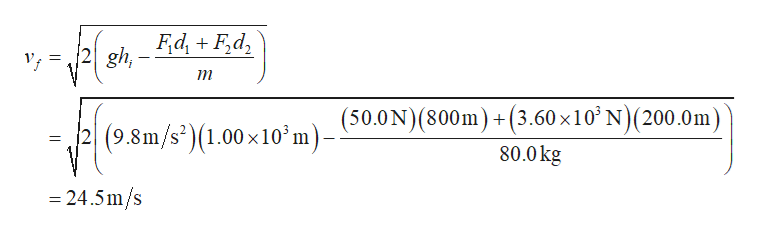# An 80.0 - kg skydiver jumps out of a balloon at an altitude of1.00 x 103 m and opens the parachute at an altitude of 200.0 m.(a) Assuming that the total retarding force on the diver isconstant at 50.0 N with the parachute closed and constant at3.60 x 103 N with the parachute open, what is the speed ofthe diver when he lands on the ground? (b) Do you think theskydiver will get hurt? Explain. (c) At what height should theparachute be opened so that the final speed of the skydiverwhen he hits the ground is 5.00 m/s? (d) How realistic is theassumption that the total retarding force is constant? Explain.

Question
3 views

An 80.0 - kg skydiver jumps out of a balloon at an altitude of
1.00 x 103 m and opens the parachute at an altitude of 200.0 m.
(a) Assuming that the total retarding force on the diver is
constant at 50.0 N with the parachute closed and constant at
3.60 x 103 N with the parachute open, what is the speed of
the diver when he lands on the ground? (b) Do you think the
skydiver will get hurt? Explain. (c) At what height should the
parachute be opened so that the final speed of the skydiver
when he hits the ground is 5.00 m/s? (d) How realistic is the
assumption that the total retarding force is constant? Explain.

check_circle

Step 1

(a). according to the work energy theorem in the entire trip

Step 2

Since the retarding force is opposite to the motion of the diver and the final potential energy and initial kinetic energy of the diver is zero. Because the diver is starting from rest and the final position is to the ground.

Step 3

Solve the above equation...help_outlineImage TranscriptioncloseF,d, + F,d, 2 gh,- т (500N)(800m) +(3.60х10' N)(200.0m) 2|(9.8m/s*)(1.00×10° m)- 80.0 kg = 24.5 m/s fullscreen

### Want to see the full answer?

See Solution

#### Want to see this answer and more?

Solutions are written by subject experts who are available 24/7. Questions are typically answered within 1 hour.*

See Solution
*Response times may vary by subject and question.
Tagged in

### Other